# Movie Plot Synopses with Tags : Tags PredictionAbstract social tagging of movies reveals a wide range of heterogeneous information about movies, like the genre, plot structure, soundtracks, metadata, visual and emotional experiences. Such information can be valuable in building automatic systems to create tags for movies. Automatic tagging systems can help recommendation engines to improve the retrieval of similar movies as well as help viewers to know what to expect from a movie in advance. In this paper, we set out to the task of collecting a corpus of movie plot synopses and tags. We describe a methodology that enabled us to build a fine-grained set of around 70 tags exposing heterogeneous characteristics of movie plots and the multi-label associations of these tags with some 14K movie plot synopses. We investigate how these tags correlate with movies and the flow of emotions throughout different types of movies. Finally, we use this corpus to explore the feasibility of inferring tags from plot synopses. We expect the corpus will be useful in other tasks where analysis of narratives is relevant.

##### Problem Statement :

Suggest the tags based on the content that was there in the Movie in its Title and Synopsis.

##### Real World / Business Objectives and Constraints :
1. Predict as many tags as possible with high precision and recall.
2. Incorrect tags could impact customer experience.
3. No strict latency constraints.
##### Machine Learning problem :

Data Overview :

All of the data is in 1 file: mpst_full_data.csv. Data is divided into 3 Sections Train, Test and Val data within the file
Train Consist of 64% of the data.
Test Consist of 20% of the data.
Val Consist of 16% of the data.
Number of rows in mpst_full_data.csv = 14828

Data Field Explanation :

Data set contains 14,828 rows. The columns in the table are:

• imdb_id — IMDB id of the movie
• title — Title of the movie
• plot_synopsis — Plot Synopsis of the movie
• tags — Tags assigned to the movie separated by “,”
• split — Position of the movie in the standard data split, like Train, Test or Val
• synopsis_source — From where the plot synopsis was collected

Example Data point :

• imdb_id: tt0113862
• title : Mr. Holland’s Opus

#### Mapping the real-world problem to a Machine Learning Problem :

##### Multi-label Classification:

Multi label classification assigns to each sample a set of target labels. This can be thought as predicting properties of a data-point that are not mutually exclusive, such as topics that are relevant for a document. A Movie might be about any Genre like romantic, action, thriller, horror at the same time or none of these.

##### Performance metric :

Micro-Averaged F1-Score (Mean F Score) : The F1 score can be interpreted as a weighted average of the precision and recall, where an F1 score reaches its best value at 1 and worst score at 0. The relative contribution of precision and recall to the F1 score are equal. The formula for the F1 score is:

F1 = 2 * (precision * recall) / (precision + recall)

In the multi-class and multi-label case, this is the weighted average of the F1 score of each class.

‘Micro f1 score’:
Calculate metrics globally by counting the total true positives, false negatives and false positives. This is a better metric when we have class imbalance.

‘Macro f1 score’:
Calculate metrics for each label, and find their unweighted mean. This does not take label imbalance into account.

##### Data Analysis :

Let us see some meta data, i.e. data about our dataset.

• Total Number of Rows : 14828
• Total Number of Columns : 6
• Columns : imdb_id, title, plot_synopsis, tags, split, synopsis_source, tag_count
• tag_count : is a newly added column that specifies how many tags our movie consist of.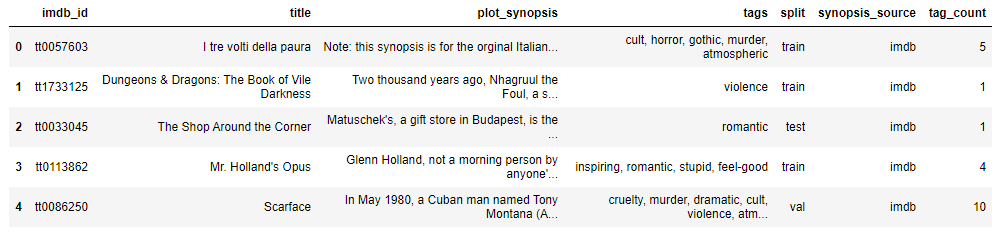##### Tags Analysis :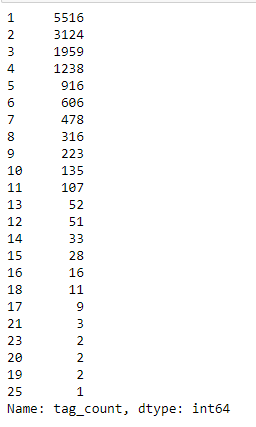We can see that there are 5516 movies with 1 tag, similarly 2 movies with 3124 tags, all the way to we have 1 movie with 25 tags in it. This gives a lot of information of the distribution of tags among the movies.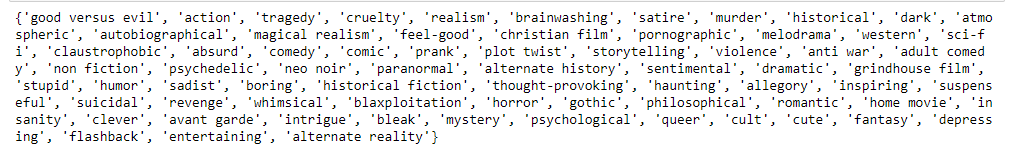As we can see there are 71 unique tags we have in our dataset, we will use these tags to predict the test data.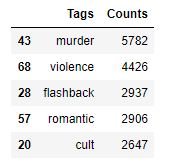We can see that “murder”, “violence”, “romance”, “flashback” and “cult” as some of the popular tags we have in the dataset. Avg. number of tags per movie: 2.794578 ~ 3 tags per movie.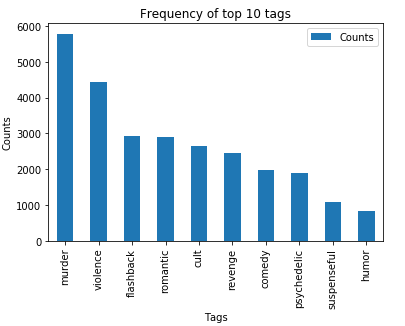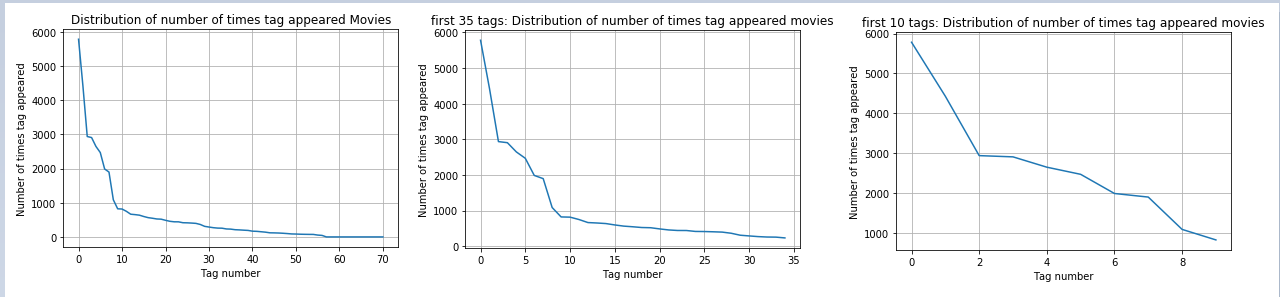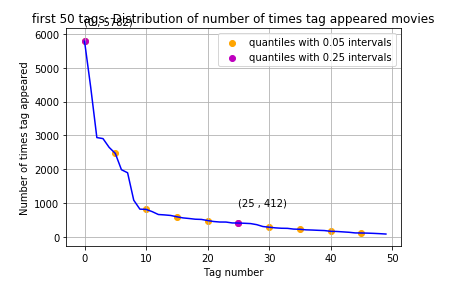##### Let’s draw our Observations over the analysis :
1. There are total 20 tags which are used more than 500 times.
2. 9 tags are used more than 1000 times.
3. Most frequent tag (i.e. murder) is used 5782 times.
4. Since some tags occur much more frequently than others, Micro-averaged F1-score is the appropriate metric for this problem.

#### Data Pre Processing :

##### Data Cleaning :

We clean the data, as in we have raw form of textual data, we remove the following :

1. punctuation
2. extra spaces
3. stop words
4. we change words like “won’t” to “will not”, “can’t” to “can not” etc.
5. we change words like “I’ve” to “I have” , “I’ll” to “I will”

After Cleaning the data it looks like :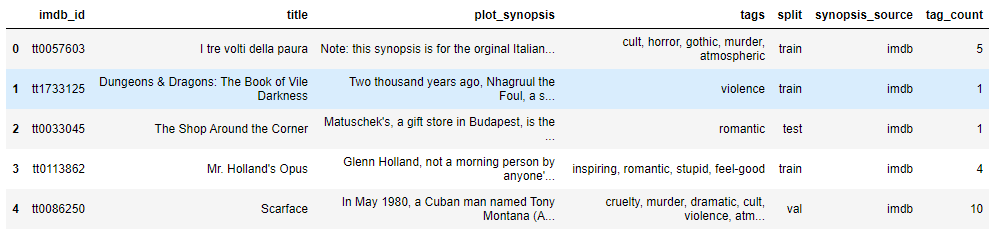#### Machine Learning Models :

##### Converting tags for multi-label problems :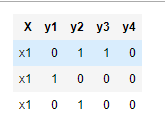We convert all the 71 tags into a binary bow, where for each movie we will put 1 against those tags which are present for the movie.

After converting all the tags into a bow feature, we have tags in the form of (14828, 71) dataset.

Split the data into test and train :

We split the data in the manner of (80:20) split. After splitting we have data points as :

Number of data points in train data X : (11862, 1)
Number of data points in train data Y : (11862, 71)
Number of data points in test data X : (2966, 1)
Number of data points in test data Y : (2966, 71)

Featurising Data : The most important part of any Model

Let us talk about the most creative and most toughest part about any Machine Learning model, i.e. creating the features out of the raw data..

Lets us look into features which i came up with while solving the problem.

##### Lexical Features :
1. n-Gram : 1,2,3
2. Char n-Gram : 2,3
3. k-Skip-n-Gram

Will Explore the Lexical Features one-by-one :

1. n-Gram : 1,2,3

We will understand this with an example : Let’s take this sentence into consideration “The quick brown fox jumps over the lazy dog”. Now for a feature of 1-gram it will consider each word to be a vector :

The, quick, brown, fox, jumps, over, the, lazy, dog

Similarly for 3-gram it will take : The quick brown, quick brown fox,… and so on.

They are basically a set of co-occuring words within a given window.

2. Char n-gram : 2,3

Character n-Gram implies the same concept as n-gram the only difference is, it works on a character level. For Example : Consider the word “Machine Learning”

• Char-2-Gram : “ma”, “ac”, ”ch”,”hi”… and so on
• Char-3-Gram : “mac”,”ach”,”chi”… and so on

3. k-Skip-n-Gram :

In skip gram architecture of word2vec, the input is the center word and the predictions are the context words. Consider an array of words W, if W(i) is the input (center word), then W(i-2), W(i-1), W(i+1), and W(i+2) are the context words, if the sliding window size is 2.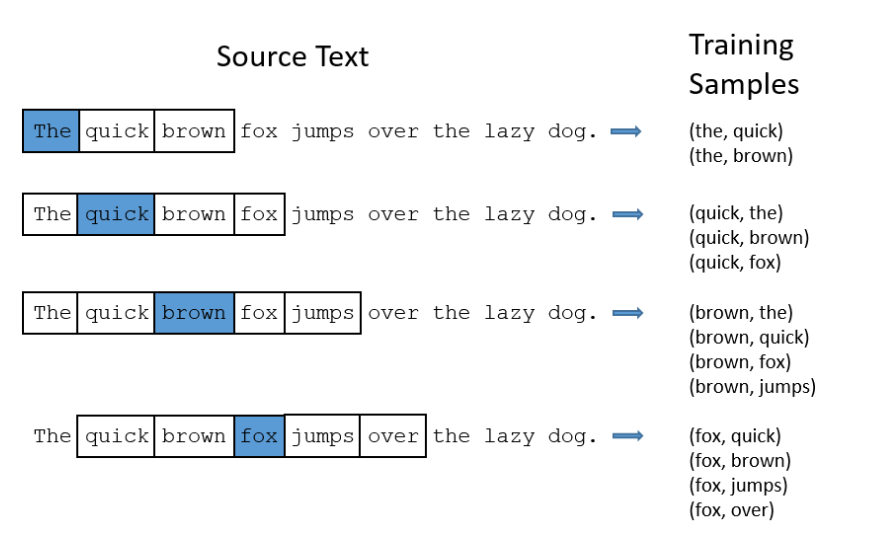As we say, picture speaks more than words, well that’s absolutely true. The above image just saved me from a lots of explanation. It is clear how k-Skip-n-Gram works.

##### Bag Of Concepts : Parts Of Speech Tagging

It is a process of converting a sentence to forms — list of words, list of tuples (where each tuple is having a form (word, tag)). The tag in case of is a part-of-speech tag, and signifies whether the word is a noun, adjective, verb, and so on.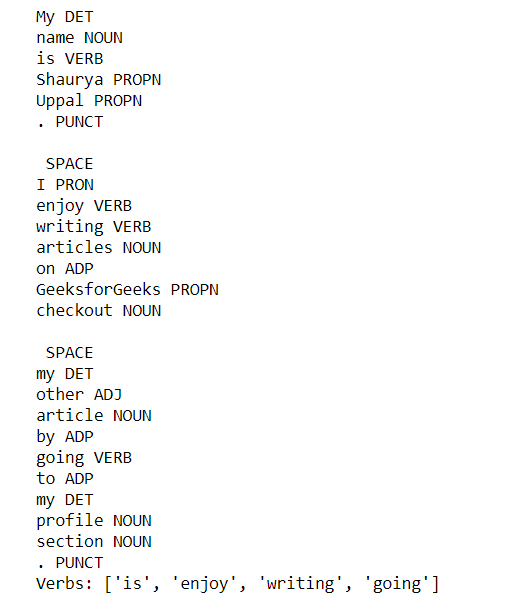Basically POS tagging is word wise, so we have to very careful before using them that how are we suppose to use them for our machine, in my case i counted the number of POS i have in my sentence and made a bow model and updated the count, this way there will be no dimension mismatch in our data.

##### Sentiments and Emotions :

I used “SentimentIntensityAnalyzer” to find out the sentiment values of a particular sentence, this function returns us with 4 dimension feature along with their value for a particular sentence, the values are basically : neg, neu, pos, compound : these are scores based on the analysis of the text given.

#### Word Embedding :

I have used 4 word Embedding, namely :

1. BOW
2. TF-IDF
3. Avg W2V
4. TFIDF weighted AvgW2V

Lets go through each one of them and understand their significance :

BOW :

The bag-of-words model is a way of representing text data when modeling text with machine learning algorithms. Machine learning algorithms cannot work with raw text directly; the text must be converted into numbers. Specifically, vectors of numbers.

A bag-of-words model, or BoW for short, is a way of extracting features from text for use in modeling. A bag-of-words is a representation of text that describes the occurrence of words within a document. For example : Let’s consider 2 sentences :

1. “This is a good place to stay”
2. “This is a good place to eat and drink”

Now, we need to have 2 things to make up our BoW model,

• A vocabulary of known words.
• A measure of the presence of known words.

So, lets us consider a something called a Binary BoW, which specifies whether that sentence contains the word or not. Here our BoW vector will be like [This, is, a, good, place, to, stay, eat, and, drink]. Hence the binary BoW for 1st and 2nd sentence will be as follows :[1,1,1,1,1,1,1,0,0,0] and [1,1,1,1,1,1,1,0,1,1] respectively.

##### TF-IDF :

Tf-idf stands for term frequency-inverse document frequency, and the tf-idf weight is a weight often used in information retrieval and text mining. This weight is a statistical measure used to evaluate how important a word is to a document in a collection or corpus. The importance increases proportionally to the number of times a word appears in the document but is offset by the frequency of the word in the corpus.

TF: Term Frequency, which measures how frequently a term occurs in a document. Since every document is different in length, it is possible that a term would appear much more times in long documents than shorter ones. Thus, the term frequency is often divided by the document length (aka. the total number of terms in the document) as a way of normalization:

TF(t) = (Number of times term t appears in a document) / (Total number of terms in the document).

IDF: Inverse Document Frequency, which measures how important a term is. While computing TF, all terms are considered equally important. However it is known that certain terms, such as “is”, “of”, and “that”, may appear a lot of times but have little importance. Thus we need to weigh down the frequent terms while scale up the rare ones, by computing the following:

IDF(t) = log_e(Total number of documents / Number of documents with term t in it).

##### Avg W2V :

Word2vec basically place the word in the feature space is such a way that their location is determined by their meaning i.e. words having similar meaning are clustered together and the distance between two words also have the same meaning.

lets first understand what is cosine similarity because word2vec uses cosine similarity for finding out the most similar word. Cosine similarity is not only telling the similarity between two vectors but it also test for orthogonality of vector. Cosine similarity is represented by formula:if angle are close to zero than we can say that vectors are very similar to each other and if theta is 90 than we can say vectors are orthogonal to each other (orthogonal vector not related to each other ) and if theta is 180 we can say that both the vector are opposite to each other.

We need to give large text corpus where for every word it creates a vector. it tries to learn the relationship between vector automatically from raw text. larger the dimension it has, larger it is rich in information the vector is.

properties:

1. if word w1 and w2 are similar than vector v1 and v2 will be closer.
2. automatic learn the relationship between words/vector.

we are looking into Male-Female graph we are observing that distance between man and woman is same as distance between king (male) and queen (woman) Not only different gender but if we look into same-gender we observe that distance between queen and woman and distance between king and man are same(king and man, queen and woman represent same-gender comparison hence they must be equal distance)

how to convert each document to vector?

suppose you have w1, w2, …wn word in one document(row). in order to convert into vector.

each word has one vector, we will convert average word2vec than divide by the number of word in a document.

##### TF IDF weighted Word2Vec :

In this method first, we will calculate tf idf value of each word. than follow the same approach as above section by multiplying tf idf value with the corresponding word and then divided the sum by sum tfidf value. Hence, these 4 techniques were used to add as a feature to my model’s training.

Numerical Feature for Text :

• Len of each Plot Synopsis : Basically, i took the length of each plot synopsis and made that as an additional feature.
• Len of Unique words in Plot Synopsis : Counted each unique word for every plot synopsis and included that as a feature.

Combining All the Hand-Made Features :

Finally after combining all the features, we have all together of 12 features to be used for Training of my model.

#### Models :

##### 1. Multi-Label Classification : Machine Learning

1.1 : One Vs Rest : Logistic Regression :

One-vs-the-rest (OvR) multi-class/multi-label strategy. Also known as one-vs-all, this strategy consists in fitting one classifier per class. For each classifier, the class is fitted against all the other classes. In addition to its computational efficiency (only n_classes classifiers are needed), one advantage of this approach is its interpretability. Since each class is represented by one and one classifier only, it is possible to gain knowledge about the class by inspecting its corresponding classifier. This is the most commonly used strategy for multi-class classification and is a fair default choice.

This strategy can also be used for multi-label learning, where a classifier is used to predict multiple labels for instance, by fitting on a 2-d matrix in which cell [i, j] is 1 if sample i has label j and 0 otherwise. In the multi-label learning literature, OvR is also known as the binary relevance method. After using OVR : LR and hyper tuning it, we got the below results :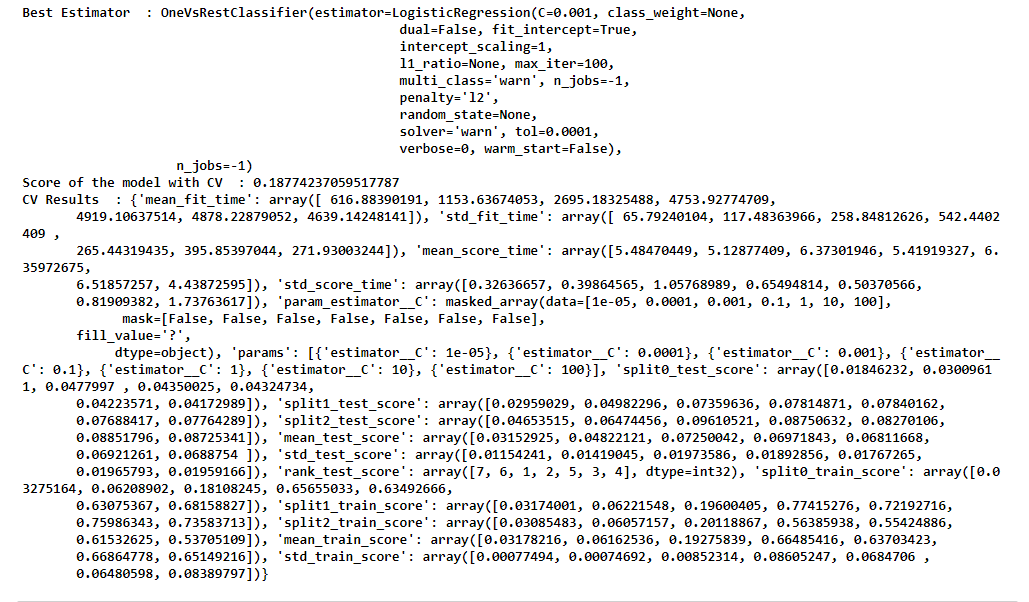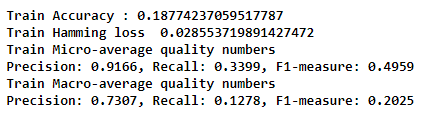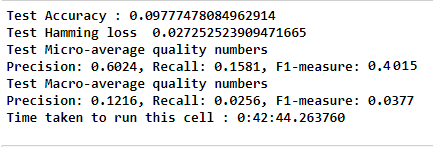Here, all the tags are given a probability for being in the plot synopsis, hence i considered only those which had a probability of more than 0.25

1.2 : One Vs Rest : MultinomialNB

I used, Multinomial Naive Bayes, as a classifier along with OVR, lets visualize the results :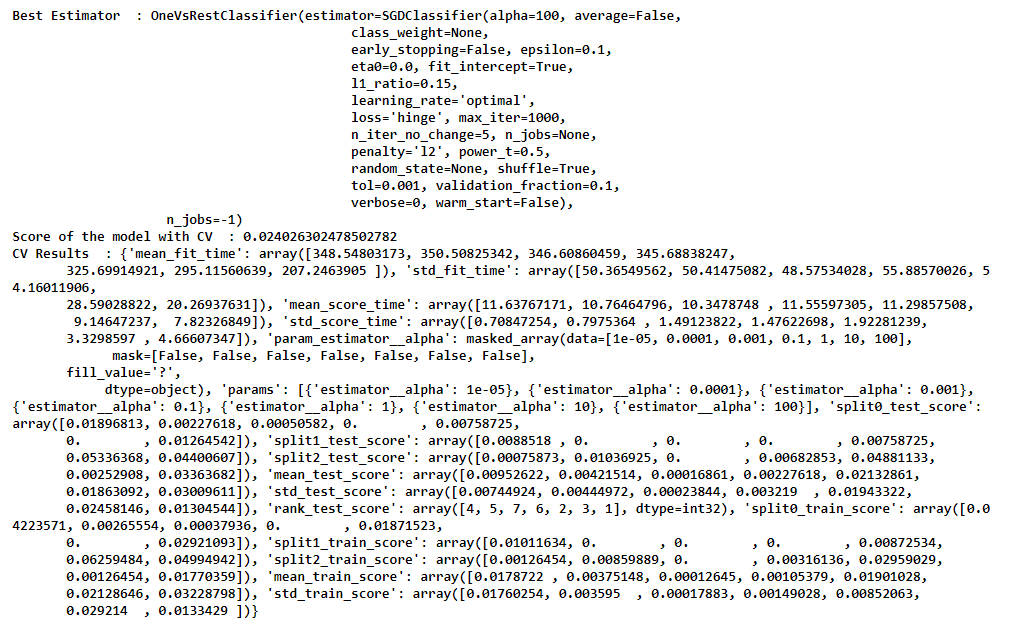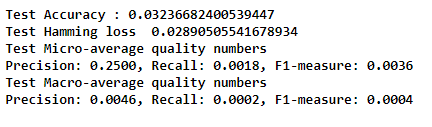Test F1 Score with prob > 0.495000 for each tags : 0.076542 . Very less, cannot be considered.

##### 2. Multi-Label Classification : Deep Learning

For Deep Learning, we need to consider, custom metrics and our custom Word Embedding.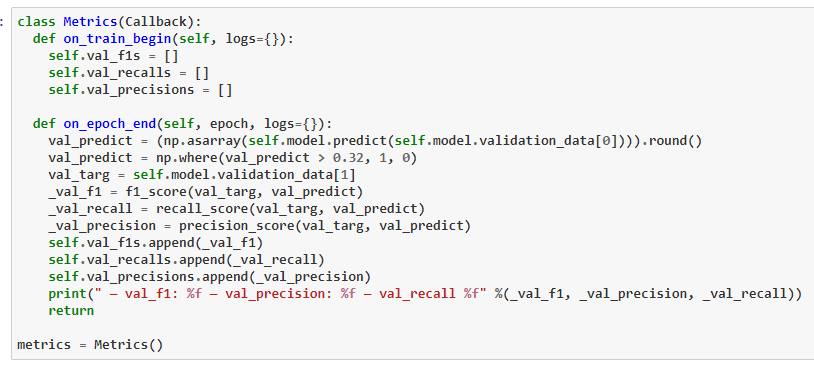Featurization :

I took the whole raw text data, and performed Word Embedding for the same. We can use Tokenizer and with Max_No_Words, Max_Seq_Length and Embedding_Dim.

In word embedding, every word is represented as an n-dimensional dense vector. The words that are similar will have similar vector. Word embedding techniques such as GloVe and Word2Vec have proven to be extremely efficient for converting words into corresponding dense vectors. The vector size is small and none of the indexes in the vector is actually empty.

To implement word embedding, the Keras library contains a layer called Embedding(). The embedding layer is implemented in the form of a class in Keras and is normally used as a first layer in the sequential model for NLP tasks.

Splitting Train and Test Data (80:20) :

I split the data into Train and Test For the DL models.

2.1 : Model 1 : Embedding + Conv1D + Conv1D + LSTM

Model Comprises of : Embedding Layer, DropoutConv1DDropoutConv1DLSTMSigmoid Layer..

Test F1 Score with prob > 0.500000 for each tags : 0.018086 The score is really not good, will have to try something else.

2.2 : Model 2 : Embedding + Conv1D + Conv1D + LSTM + LSTM

This model consists of the Following sequence :

Embedding > Conv1D > Dropout > Conv1D > LSTM > BatchNorm > LSTM > Sigmoid

References links for the explanations have already been provided in Model 1, I have mentioned BatchNorm explanation link in the sequence.

Test F1 Score with prob > 0.250000 for each tags : 0.296534. Still the F1 score cannot be considered to be as good.. Let’s Move on.

2.3 : Model 3 : Embedding + Conv1D + BN + LSTM

Here, embedding means, i did a custom Embedding using Glove Vector, and used those embedding to train and predict the test data. As, per the Above explanations, we all know about the elements of the models, lets dive into the results :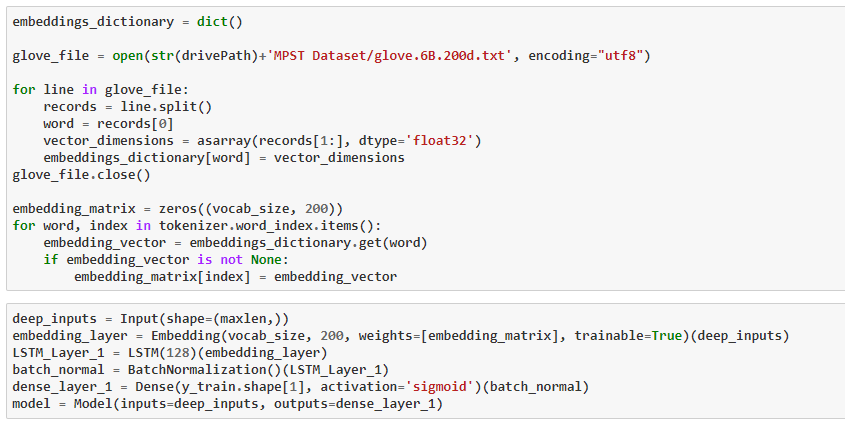So the F1-score is 0.34 which is better than above, can be considered, but lets see can we get more than that..

2.4 : Model 4 : Embedding + Conv1D + Conv1D + LSTM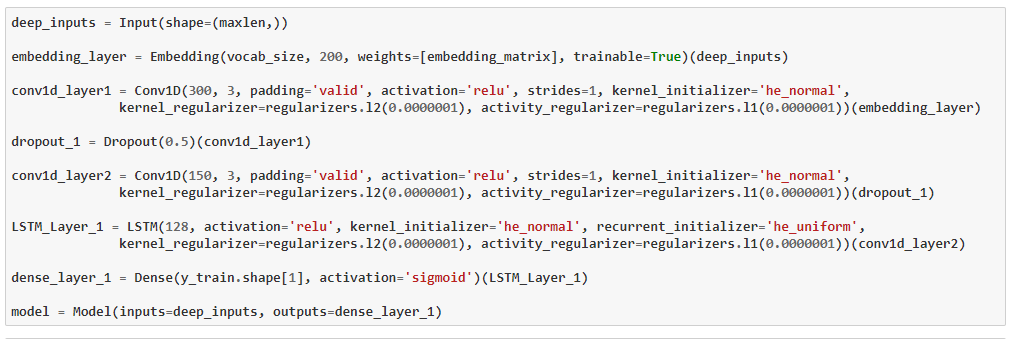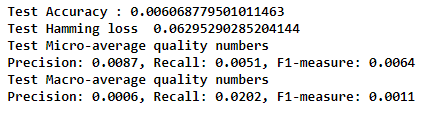Worst model ever, Lets try one more model.

2.5 : Model 5 : Embedding + Conv1D + BN + Conv1D + BN + LSTM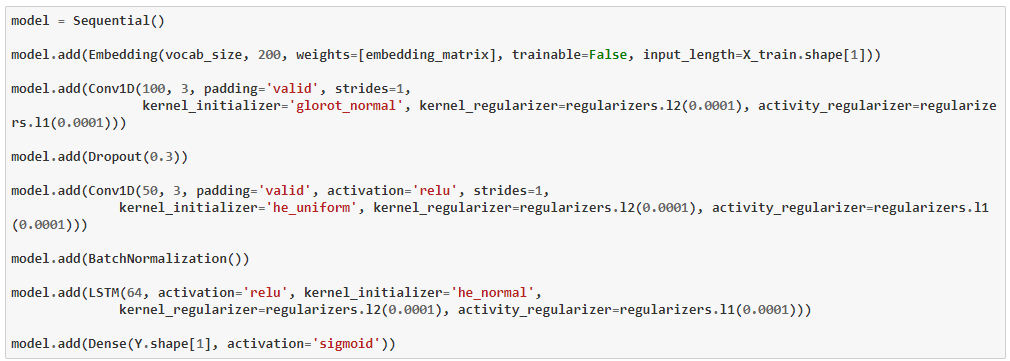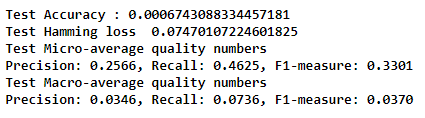Test F1 Score with prob > 0.150000 for each tags : 0.330111

After trying, so many models, it seem we need some more fine tuning the models, and come up with more than features. Lets summarize out results, and give a closure conclusion to this problem.

##### Model Summary :

Machine Learning :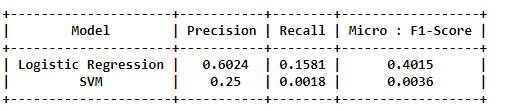Deep Learning :##### Conclusion :
1. The maximum micro averaged F1 score is 0.4015 and the maximum value of recall is 0.4827.
2. Char N-gram features proved to be significantly powerful than word N-gram features. Skip Grams were also useful.
3. Using featurization like bow, avg word2vec, tfidf word2vec and combination of TF-IDF and Word2Vec features, our models behaved surprisingly better than the previous implementation.
4. In today’s era, we are more used to see scores above 90%. But given a very limited data size sample of 14K datapoints, we have actually managed to get a decent micro averaged F1 score.
##### Further Improvements :

We can use more features into Deep Learning models, some more fine tuned architecture, more hyper tuning can be done. We can achieve a good f1 score when we add more features, and consider a deeper network.

##### References :Hello ! I am Bishal Bose. You can mail me at bishalbose294@gmail.com if you have any queries regarding the project. Connect with me on instagram. Click here